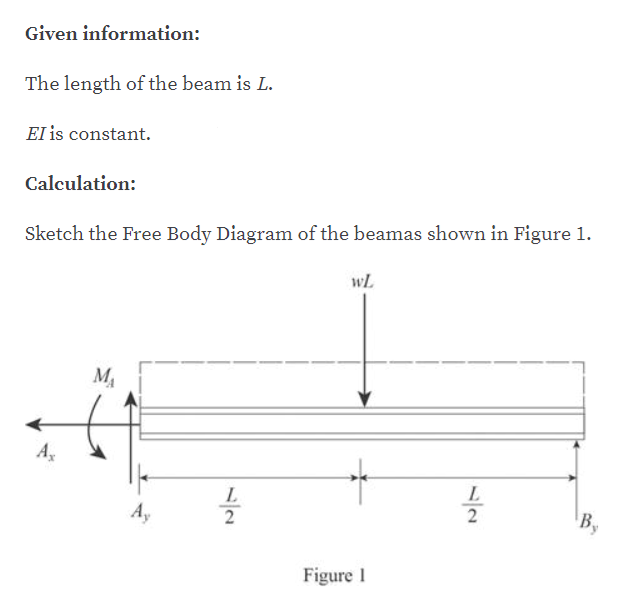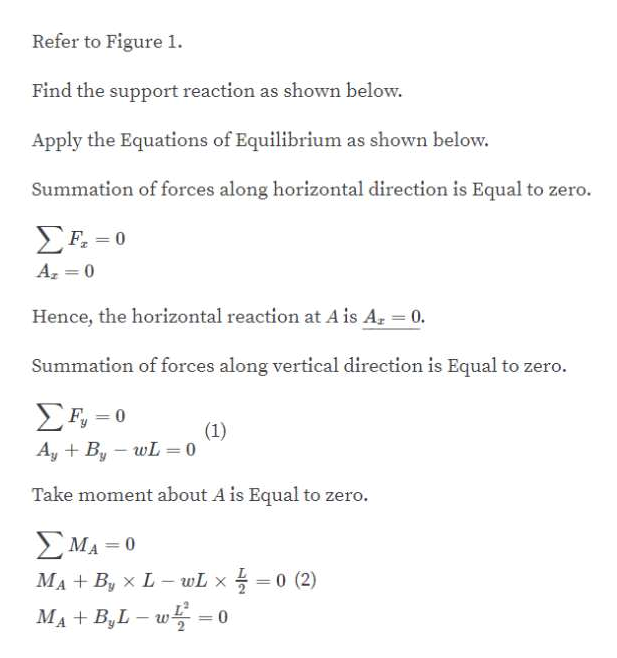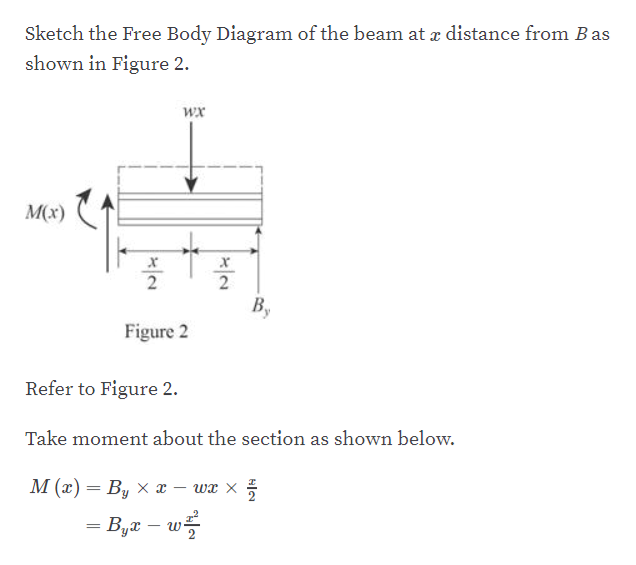# AL_

Question

determine the reactions at the supports A and B, then draw the shear and moment diagrams. EI is constant.

check_circleExpert Solution
Step 1help_outlineImage TranscriptioncloseGiven information: The length of the beam is L. EI is constant Calculation: Sketch the Free Body Diagram of the beamas shown in Figure 1 wL М L L 2 2 В, Figure fullscreen
Step 2help_outlineImage TranscriptioncloseRefer to Figure 1 Find the support reaction as shown below Apply the Equations of Equilibrium as shown below. Summation of forces along horizontal direction is Equal to zero. ΣΗ-0 F 0 A 0 Hence, the horizontal reaction at A is A 0. Summation of forces along vertical direction is Equal to zero. Ση-0 (1) AyBy wL= 0 Take moment about A is Equal to zero. ΣΜΑ 0 0 (2) MABy x L- wL x MA+B,L- 0 fullscreen
Step 3help_outlineImage TranscriptioncloseSketch the Free Body Diagram of the beam at x distance from B as shown in Figure 2 wx Mx) x x 2 В, Figure 2 Refer to Figure 2. Take moment about the section as shown below. М (") — В, х а — wx х _ fullscreen

### Want to see the full answer?

See Solution

#### Want to see this answer and more?

Solutions are written by subject experts who are available 24/7. Questions are typically answered within 1 hour*

See Solution
*Response times may vary by subject and question
Tagged in

### Solid Mechanics